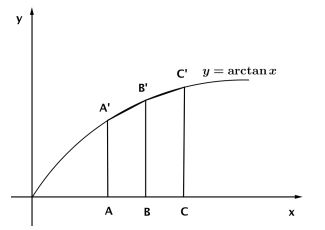# Dan Sitaru's Exercise with Pi and Ln

### Problem### Solution$A(x,0); B(y,0); C(z,0)\\ A'(x,\arctan x); B'(y,\arctan y); C'(z,\arctan z)$

Let $[X]\,$ denote the area of shape $X.\,$ Using the fact that $arctan(x)\,$ is a concave function,

\displaystyle \begin{align} &[ABB'C']+[BCC'B']\lt \int_0^1 \arctan x dx\\ &\frac{(AA'+BB')(y-x)}{2}+\frac{(BB'+CC')(z-y)}{2}\lt\int_0^1 \arctan x dx\\ &\frac{(\arctan x+\arctan y)(y-x)}{2}+\frac{(\arctan y+\arctan z)(z-y)}{2}\\ &\qquad\qquad\qquad\qquad\lt \left(x \arctan x-\frac{1}{2}\ln(x^2+1)\right)\Bigr|_0^1\\ &(y-x)\arctan x+(y-x+z-y)\arctan y+ (z-y)\arctan z\\ &\qquad\qquad\qquad\qquad\lt 2\cdot\left(\frac{\pi}{4}-\frac{1}{2}\ln 2\right)\\ &(y-x)\arctan x+(z-x)\arctan y+(z-y)\arctan z\lt \frac{\pi}{2}-\ln 2 \end{align}

### Acknowledgment

Dan Sitaru has kindly mailed me everything needed to put together a web page: a pdf file for easy read, a LaTeX file for easy write, a graphics file to grasp at a glance, and a GeoGebra file to produce or easily modify the graphics. I appreciate that very much.